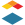# [New Quizzes] Strict Decimal Places and Significant Figures in Numeric and Formula Questions

Problem statement:

Numeric quiz questions allow one to choose "Precise response" and then choose "Decimal places" or "Significant figures" for the answer. Formula quiz questions allow one to chose "Decimal places" for the answer. In the current implementation trailing 0s are ignored. Examples- -> If one decimal place is chosen and 3.4 is the correct answer, then 3.40 will also be marked as correct. -> If two significant figures are chosen and 3.4 is the correct answer, then 3.40 will also be marked as correct. I understand why this is useful in math classes and other applications. But, in chemistry it can be a problem since decimal places deal with measurement uncertainty and if a piece of equipment can only be read to one decimal place then two decimal places is not correct. We also have questions which involve significant figures and if the final answer should only have two significant figures then 3.40 has three and is not correct. As others have posted in at least one thread, one can hard-code the possible answers as text and then specify a number of alternate choices and this is how we do it. But this leaves out formula questions which would be useful to use. Hard-coding is time-intensive and in large classes a small number of choices means more opportunities for students to share information.

Proposed solution:

I propose an additional choice of "strict" decimal places and "strict" significant figures. This would allow one to choose whether student answers with trailing 0s should or should not be ignored. If trailing 0s are not an issue, then the current implementation works. If trailing 0s are important, then the additional choice of "Strict" (or something similar) would be very useful.

User role(s):

instructor,designer